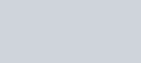A man walks a certain distance with certain speed.
Question:

A man walks a certain distance with certain speed. If he walks 1/2 km an hour faster, he takes 1 hour less. But, if he walks 1 km an hour slower, he takes 3 more hours. Find the distance covered by the man and his original rate of walking.

Solution:

Let the actual speed of the train be x Km/hr and the actual time taken by y hours. Then,

Distance covered= speed $\times$ dis tance

$=x \times y$

$=x y \ldots(i)$

If the speed is increased by $\frac{1}{2} \mathrm{Km} / \mathrm{hr}$, then time of journey is reduced by 1 hour i.e., when speed is $\left(x+\frac{1}{2}\right) \mathrm{km} / \mathrm{hr}$, time of journey is $(y-1)$ hours

Distance covered $=x y \mathrm{~km}$

$x y=(x-1)(y+3)$

$x y=(x-1)(y+3)$

$x y=x y-1 y+3 x-3$Thus we obtain the following equations

$-2 x+1 y-1=0$

$3 x-1 y-3=0$

By using elimination method, we havePutting the value $x=4$ in equation (iii) we get

$3 x-1 y-3=0$

$3 \times 4-1 y-3=0$

$12-1 y-3=0$

$12-3-1 y=0$

$9-1 y=0$Putting the value of and y in equations (i) we get

Distance covered $=x y$

$=4 \times 9$

$=36 \mathrm{~km}$

Hence, the distance is $36 \mathrm{~km}$,

The speed of walking is $4 \mathrm{~km} / \mathrm{hr}$.